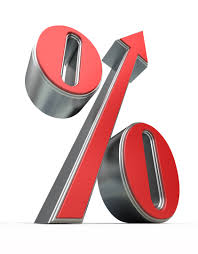# Percents, So Many Percents

Algebra Level 2What is the value $10$% of $20$% of $30$% of $40$% of $50$% of $60$% of $70$% of $80$% of $90$% of $100$?

×